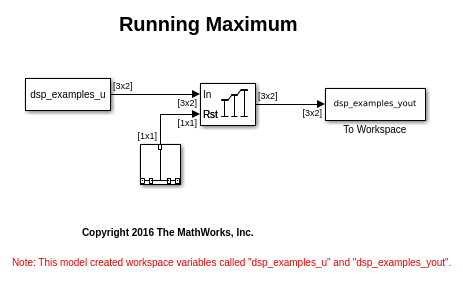Documentation

## Compute the Running Maximum

Compute the running maximum of a 3-by-2 matrix input, `dsp_examples_u`, using the Maximum block.

Open the model.

```model = fullfile(matlabroot,'examples','dsp','ex_runningmaximum_ref'); open_system(model) ```The Input processing parameter is set to `Columns as channels (frame based)`. The block processes the input as a two-channel signal with a frame size of three. The running maximum is reset at t = 2 by an impulse to the block's Rst port.

Run the model.

```sim(model) ```In the `Running` mode, the block outputs the maximum value over each channel since the last reset. At t = 2, the reset event occurs. The maximum value in the second column changes to 6, even though 6 is less than 9, which was the maximum value since the previous reset event.

Close the model.

```close_system(model) ```